Technical Information Site of Power Supply Design

• TECH INFO
• What capacitor and inductor are the best for a switching power supply? - Capacitor - : In the evaluation of output ripples, ESL for output capacitor requires particular attention

Ask Direct to Engineers

What capacitor and inductor are the best for a switching power supply?

# In the evaluation of output ripples, ESL for output capacitor requires particular attention

## Capacitor – Part 4 –

Keyword MLCCFunctional polymer capacitorOutput capacitorRipple voltageESRESL

－ Please tell us about required performance characteristics when the product is used as an output capacitor, and the impact of its properties.

As I mentioned before, it goes without saying that in a switching power circuit, output capacitors, as in the case of input capacitors, represent critical components. As in the case of the input capacitor, the impact of parasitic components, such as ESR and ESL in addition to electrostatic capacitance, need to be taken into consideration. That said, the output capacitor differs from the input capacitor in that the current waveform applied is different and that it has a load to be contended with. Consequently, the voltage fluctuations and phenomena that occur are different. At any rate, the issue is how to minimize the voltage fluctuations in the output. Specifically, the output ripple voltage and the load transient response must be addressed.

－ Then, could you talk about the output ripple voltage? Given the fact that a current flows different from the input, it would be helpful if you started with the current that flows in the output capacitor, as you described in the case of the input capacitor.

Let us take an example of a step-down converter. First, look at the power supply circuit diagram and the figure that illustrates the waveform components of ripples. The switching of the output-stage transistor generates a triangular inductor current, with the result that the average current for the inductor indicated as “DC” flows to the output, and the triangular component of the current flows as AC to the output capacitor. The waveform diagram below shows what kind of voltage is generated by the triangular capacitor current, depending upon the ESL and ESR, and upon the capacitance components.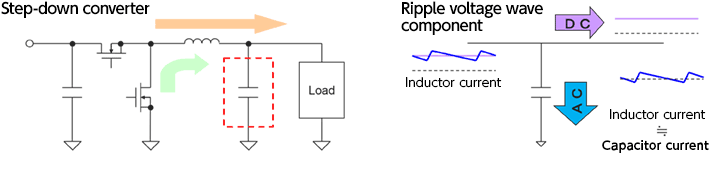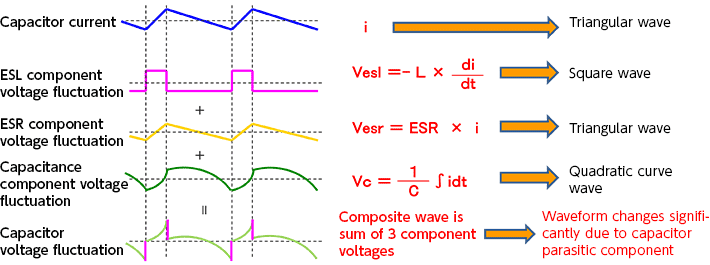The capacitor current of a triangular wave manifests itself as a square wave voltage due to ESL. Depending on the ESR the capacitor current directly becomes a triangular wave voltage according to Ohm’s Law, and based on its capacitance it acquires a time constant. Ultimately, the capacitor current turns into a composite wave of these three components. Simply stated, the sharp rise is due to the ESL, the fluctuations with a slope are due to the ESR, and each as a function of capacitance undergoes changes in the form of a quadratic curve.

The waveform diagram shown below represents an actual ripple voltage waveform. From the behavior of the waveform, you can tell which part depends on which part. This fact, which is a key factor in improving such ripples, should be borne in mind.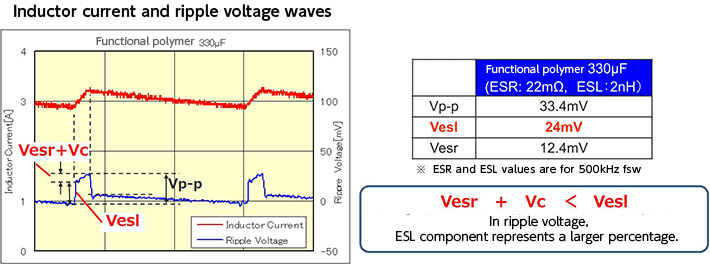The top waveform is an inductor current. The bottom one is the ripple voltage that is generated in the output. The sharp rise is due to the Vest which is produced by the ESL. The subsequent increase is the Vesr due to the ESR. The sum of these factors is the peak Vp-p of the ripple voltage. In this case, the output capacitor is a functional polymer type with a capacitance of 330 µF. ESR is 22 mΩ and ESL 2 nH. Both represent values with a switching frequency of 500 kHz. The table also shows actual voltage fluctuations. As indicated in red, Vesl is almost double the level of Vesr, representing two-thirds of the total ripple voltage peak Vp-p. These numerical values, subject to change due to frequencies and other conditions, are only examples to illustrate the point.

－ So, ESL has a larger impact? It is often said that because ripples in the output voltage depend on ESR, output capacitors with low ESR levels are favored.

That would be the correct understanding. In this example also, Vesr with respect to ESR arises. Under some conditions, however, Vesl represents a large proportion in the ripple voltage.

－I now understand the influences of ESR and ESL. That said, what impact does the capacitance exert?

This is data showing the relationship between capacitance and ripple voltage. The capacitors are functional polymer capacitors with capacitance values of 330 µF, 220 µF, and 150 µF. As the waveform diagram and the table indicate, there is little change in Vesl, indicating that Vesl is virtually independent of capacitance.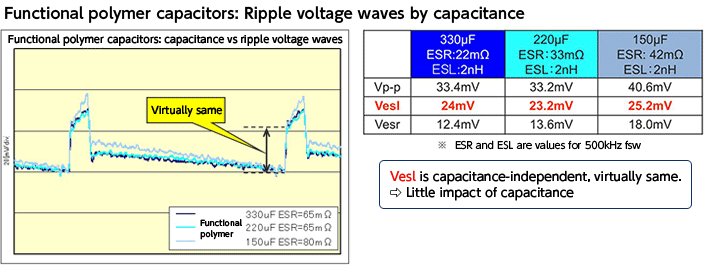－Does that mean that basically capacitors with small ESR and ESL values should be used?

Yes, indeed. As I explained previously, a characteristic of the multilayer ceramic capacitor (MLCC) lies in low ESR and low ESL. The MLCC provides a powerful element to reduce the ripple voltage, and this is the factor that is driving the recent increase in MLCCs used as the main input/output capacitors. The waveform figure below is a graph comparing the ripple voltages of functional polymer type capacitors with MLCCs, and a graph in which impedance performance characteristics are superposed on the inductor current spectrum.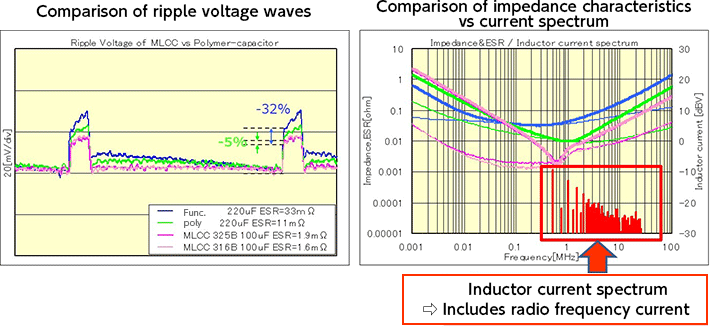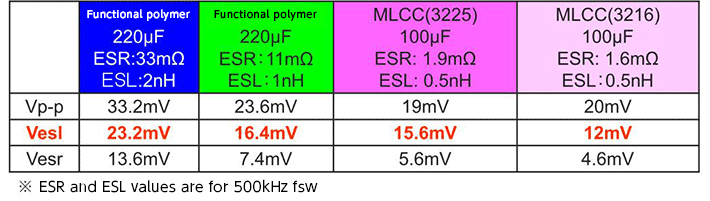In the ripple voltage waveform, the Vesl of the MLCC indicated in pink is clearly low. With respect to Vesr also, it is clear that the slope is small. As indicated by the impedance characteristic graph and the numerical values in the table, both ESR and ESL are also obviously small. In the impedance characteristic graph, the thin line represents ESR.

In this example, since the switching frequency is 500 kHz, it is evident that given the 500 kHz fundamental wave, the inductor current spectrum includes harmonics. Because that region contains frequencies that are higher than the resonance point, it is clear that ESL has a significant impact.

Also, the figure shows the relationship with respect to capacitance, in terms of mapping.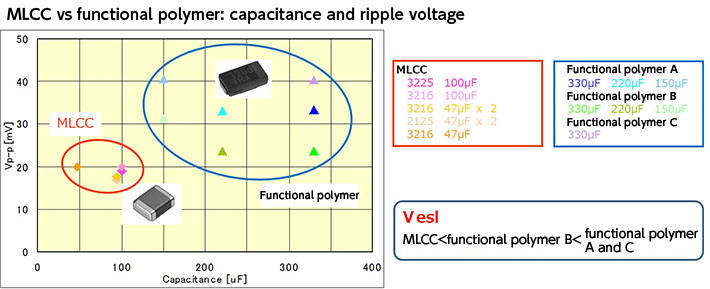The mapping indicates that the MLCC can be an extremely powerful tool in reducing the ripple voltage Vp-p and that there is hardly any dependency of Vesl on capacitance. Incidentally among the three representative brands of functional polymer type, the data suggests that there is little difference in Vesl in terms of capacitance, but there are differences in ESL by brand.

－ Looking at the comparison, I noticed that if a MLCC is to substituted for a functional polymer capacitor, these capacitors do not need to be of the same capacitance?

That is a very important point. From a ripple voltage standpoint, the MLCC offers ESR and ESL values that are both small. For a given functional polymer type, substitution can be made with a lower capacitance. The capacitance has an impact not only on size, but also on cost. Depending on the conditions to be contended with, the use of MLCCs can reduce size and cost while reducing the ripple.

## Power Supply Design Technical Materials Free DownloadThis website uses cookies.

By continuing to browse this website without changing your web-browser cookie settings, you are agreeing to our use of cookies.